Percent Word Problems
 Home > Lessons > Percent Word Problems Search | Updated November 25th, 2018
Introduction

This lesson will inform you how to solve percent word problems or problems that contain percents. Here are the sections within this page:

To fully understand the sections that follow, it is crucial that you understand how to solve equations and how to work with ratios (otherwise known as fractions). Use these lessons if you need to prepare yourself.esson: Fractionsesson: Solving Equations

Percents are used in a variety of capacities. Here are some common areas:

 Common Areas Where Percents are Found Nutrition Determining daily content for calorie, sodium, and fat intake. Shopping Finding sale price, tax, or tip amount (restaurants). Sales Calculating the amount of money made from commission. Value Adjusting the value for an item, either appreciation or depreciation

This is an important question to consider: "What is a percent?" Let us examine the word 'percent.' The word literally means per 100 because cent means 100. So, a percent is a ratio.

For instance, 50% means 50 per 100. But, 50/100 is the same as 1/2. Therefore, 50% is equivalent to a half.

To gain a visual on what 50% looks like, the glass below is at 50% or 1/2 capacity.Likewise, 75% means 75/100, which reduces to 3/4. So 75% = 3/4.

Now that you have an idea of what percents mean, we need to look at the word problem portion of percent word problems.

There are certain words that translate to mathematics. Let it be known, this is not always be true. Examine the table below to see the conversion between words and mathematics for the problems that will follow.

 Conversion: Words to Mathematics what x is = of multiply percent over 100

When we see either the words 'what,' 'is,' 'of,' or 'percent,' we will substitute their mathematical meaning into an equation. Then, we will solve the equation. Use the three types of problems below to see how it is done.

There are several exceptions to this rule, which will be handled within the section called, The Meaning of Words in Mathematics.

A type 1 problem could look like this.

"What number is 15% of 60?"

To solve this problem, we need to convert the problem from words into mathematics. Here is a word to mathematics translation.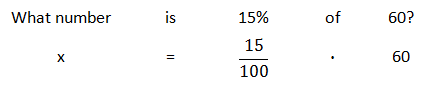To solve the resulting equation, we progress as follows.Now, we need to reduce the resulting fraction (or simplify with a calculator).So, 9 is 15% of 60.ideo: Percent Word Problemsuiz: Percent Word Problems

Here is a second type of word problem.

"20 is 30% of what number?"

Again, we need to convert this problem into mathematics. Here is the translation.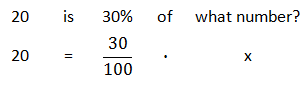Now, we will solve this equation.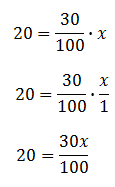To solve this equation, we have to multiply the coefficient of the x-term by its reciprocal. This means both sides have to be multiplied by this quantity, like so.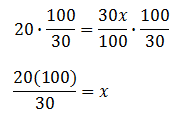Now, we need to reduce the fraction (or use a calculator) to get our final answer.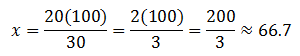So, 20 is roughly 30% of 66.7.ideo: Percent Word Problemsuiz: Percent Word Problems

Here is the last type of word problem.

"40 is what percent of 500?"

This sentence needs to be converted into mathematics, like so.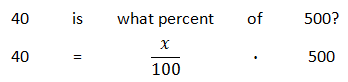Now, we will solve the resulting equation.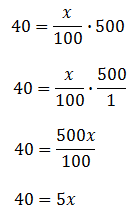To further solve this equation, we need to divide both sides by 5.So, 40 is 8% of 500.ideo: Percent Word Problemsuiz: Percent Word Problems

The lesson above is a very narrow view of percent word problems. In reality, language does not translate so nicely to mathematics. Here are some examples of the non-routine nature of language and math conversion.

1. How can 25 of 100 be represented?

This problem is asking us to compare to numbers. This can be done as a ratio, which by definition is a comparison between two numbers. So, this comparison could be written as 25/100. It could also be reduced to 1/4.

We could also represent this ratio visually. See our video Division by Zero to see how it can be done.

2. Luis felt he played his trumpet well for 30 or his last 50 performances. If he plays at the same performance rate, how many performances will Luis play well if he plays for 10 more performances?

This is another comparison of numbers (called a ratio). If we represent the ratio of 30:50 as 30/50, we could reduce it to 3/5. This means 3 out of every 5 performances Luis plays well. This is Luis' success rate.

Continuing with this problem, we now have to determine how well Luis will play out of the next ten performances. 3/5 is equal to 6/10. So, Luis will likely play 6 performances well out of his next 10 performances.

3. Six out of every 8 pairs of socks had holes with one sock. One out of 8 pairs of socks had holes with both socks. If a sock is chosen at random, what is the likelihood of it having a hole?

The problem with these socks is that we first look at single socks and then we compare pairs of socks. This mismatch needs to be fixed.

"Six out of every 8 pairs of socks had holes with one sock" means there are really 16 socks and 8 have holes in them.

"One out of 8 pairs of socks had holes with both socks" means two socks have holes for every 16 socks.

Now that we are talking about the same thing, we can put these ideas together into a single statement. This means there are 10 socks that have holes for every 16 socks. So, if a sock is chosen randomly among the 16 socks, the probability of getting one with a hole is 10/16. This ratio is equal to 5/8 or 0.625 or 62.5% (see Fractions, Decimals, and Percents for more information on how to convert between the three).

It can be seen how mathematics can be used to solve problems. The key for solving these problems is understanding what the problems means. So, the notion that all problems fit into an easily converted algorithm is not true.

However, the main lesson on this page is designed to give a student the skills to deal with a simple set of conditions. By understanding the simple set of conditions, a student can then add those skills into a growing knowledge base and likely be able to solve a greater number of problems.

Try this instructional video to gain a more personalized view on this topic.ideo: Percent Word Problems

Try this interactive quiz to test your knowledge of percent word problems.uiz: Percent Word Problems